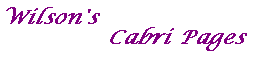#### Proof of Theorem 1

Theorem 1
If C: xTMx=0 is a non-degenerate conic and U is any point on C,
then the algebraic polar of U with respect to C is the tangent to C at U .

Proof
Let U=[u], so that the algebraic polar is L: uTMx=0.
Suppose that L cuts C again at V=[v].
As U lies on C, uTMu=0.
As V lies on C, vTMv=0.
As V lies on L, uTMv=0.
Transposing this, and using the fact that M is symmetric, vTMu=0.
Then, by expanding the left hand side, we see that
for any real a and b, (au+bv)TM(au+bv)=0.
Thus W=[au+bv] lies on C.
If U and V are distinct, then C contains the collinear points U, V and W,
but then C would be degenerate.
Thus U=V, so that L meets C just once, and hence is a tangent.